Ch 1. Particle General Motion Multimedia Engineering Dynamics Position,Vel & Accel. Accel. varyw/ Time Accel. Constant Rect. Coordinates Norm/Tang. Coordinates Polar Coordinates RelativeMotion
 Chapter - Particle - 1. General Motion 2. Force & Accel. 3. Energy 4. Momentum - Rigid Body - 5. General Motion 6. Force & Accel. 7. Energy 8. Momentum 9. 3-D Motion 10. Vibrations Appendix Basic Math Units Basic Equations Sections Search eBooks Dynamics Fluids Math Mechanics Statics Thermodynamics Author(s): Kurt Gramoll ©Kurt GramollDYNAMICS - THEORY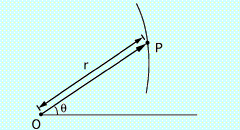Position in Polar Coordinates

For plane motion, many problems are better solved using polar coordinates, r and θ. This requires the development of position, velocity and acceleration equations based on, r and θ.

Position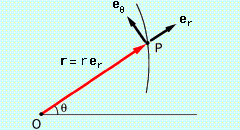Units Vectors for Polar Coordinates

Before velocity and acceleration can be determined in polar coordinates, position needs to be defined. Unlike rectilinear coordinates (x,y,z), polar coordinates move with the point and can change over time. Even though the r coordinate is moving, the position vector r, is measured in the r direction, giving
 r = r er

Velocity

Similar to other coordinate systems, velocity can be determined by taking a time derivative of position,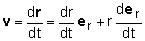Since the coordinate system is moving, the time derivative of the unit vector, er, is not zero. Using a derivation similar to that found in the theory of n-t coordinate systems, expressions for the derivatives of the unit radial and unit transverse vectors can be determined as,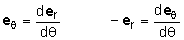The derivative of the eθ unit vector includes a negitve sign due to it changing inward as it moves (inward is a negative r-direction). The chain rule can then be used to express the time derivative of the unit radial vector as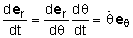Substituting the above relationships into the velocity equation gives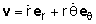Acceleration

A time derivative of the velocity will give an expression for the acceleration,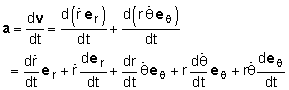The chain rule can be used to express the time derivative of the unit transverse vector as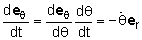By substituting this into the previous equation, and rearranging, gives the acceleration in terms of radial and transverse components,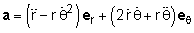It is important to note that both r and θ directions have multiple terms. Acceleration in the r and θ direction is not just d2r/dt2 and d2θ/dt2, respectively, since the coordinates are moving.

Practice Homework and Test problems now available in the 'Eng Dynamics' mobile app
Includes over 400 problems with complete detailed solutions.
Available now at the Google Play Store and Apple App Store.